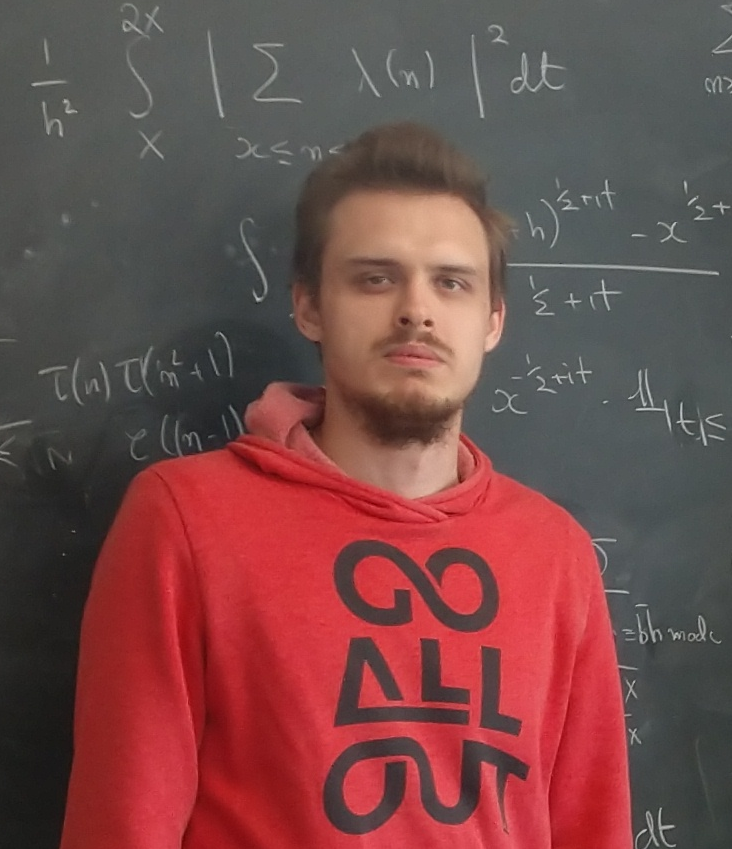## Andrei Shubin

Institute of Discrete Mathematics and Geometry
Vienna University of Technology
Freihaus, Tower A, 5th floor,
room DA 05 G02,
Wiedner Hauptstr. 8-10,
A-1040 Wien, Austria

E-mail:
name.surname at tuwien dot ac dot at

I am currently a postdoctoral researcher in the French-Austrian project ArithRand

I completed my PhD at Caltech under the supervision of Maksym Radziwiłł

Previously I was supervised by Maxim Korolev at MIPT and Steklov Mathematical Institute

I am interested in various topics at the intersection of number theory, harmonic analysis and probability

Here you can find my CV (last update in April 2023)

## Papers

M. Radziwiłł, A. Shubin, Poissonian pair correlation for $\alpha {n}^{\theta }$, preprint

A. Shubin, Möbius orthogonality of Thue-Morse sequence along Piatetski-Shapiro numbers, submitted

J.-M. Deshouillers, M. Drmota, C. Müllner, A. Shubin, L. Spiegelhofer, Synchronizing automatic sequences along Piatetski-Shapiro sequences, Israel J. Math., to appear

A. Shubin, Variance estimates in Linnik's problem, IMRN (2022),   arXiv:2108.00726,

A. Shubin, Fractional parts of non-integer powers of primes. II, Q.J.Math, 73 (1), pp. 277--310, 2022,   arXiv:2011.11790,

A. Shubin, Fractional parts of non-integer powers of primes, Math. Notes 108 (3), pp. 394--408, 2020,   arXiv:2010.15216,

A. Shubin, Bounded gaps between primes of special form, Dokl. Mathematics 101 (3), pp. 235--238, 2020

M.Korolev, A.Shubin, The second moment of the first derivative of Hardy's Z-function, Trigonometric Sums and Their Applications, Springer Nature Switzerland AG, pp. 169--182, 2020

A. Shubin, On the asymptotic behavior of a functions Ω(k; n) and ω(k; n) related to the number of prime divisors, Discrete Math. Appl. 29 (2), pp. 121--129, 2019

A. Shubin, Fractional parts of the function x/n, Math. Notes 100 (5-6), pp. 731--742, 2016

## Other

A short talk on the connection between primes and exponential sums from SSANT2021